Processing ......FreeComputerBooks.com Links to Free Computer, Mathematics, Technical Books all over the World

Theory of Computation and Computing
Related Book Categories:
 Quantum Computing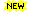Introduction to Computer Science Algorithms and Data Structures Numerical Analysis and Scientific Computing Computational and Algorithmic Mathematics Mathematical Logic - Computability, etc. Computational Complexity Computer Programming Discrete and Finite Mathematics Mathematical and Computational Software
•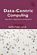A Data-Centric Introduction to Computing (Kathi Fisler, et al)

This book is an introduction to computer science. It will teach you to program, and do so in ways that are of practical value and importance. It uses a data-centric approach: data centric = data science + data structures.

•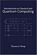Introduction to Classical and Quantum Computing (Tom Wong)

This book is for students who want to learn quantum computing beyond a conceptual level, but who lack advanced training in mathematics. The only prerequisite is trigonometry, and mathematics beyond that will be covered.

•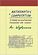Mathematics and Computation (Avi Wigderson)

This book provides a broad, conceptual overview of computational complexity theory - the mathematical study of efficient computation. With important practical applications to computer science and industry, computational complexity theory, etc.

•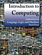Introduction to Computing: Language, Logic, and Machines

This book focuses on how to describe information processes by defining procedures, how to analyze the costs required to carry out a procedure, and the fundamental limits of what can and cannot be computed mechanically.

•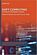Soft Computing: Techniques in Engineering Sciences

Soft Computing is used where a complex problem is not adequately specified for the use of conventional math and computer techniques. This book elaborates on the most recent applications of Soft Computing in various fields of engineering.

•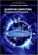Quantum Computing: Progress and Prospects

Provides an introduction to the Quantum Computing, including the unique characteristics and constraints of the technology, and assesses the feasibility and implications of creating a functional quantum computer capable of addressing real-world problems.

•Models of Computation: Exploring the Power of Computing

It covers the traditional topics of formal languages, automata and complexity classes, as well as an introduction to the more modern topics of space-time tradeoffs, memory hierarchies, parallel computation, the VLSI model, and circuit complexity.

•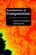Foundations of Computation (Carol Critchlow, et al)

It includes topics from discrete mathematics, automata theory, formal language theory, and the theory of computation, along with practical applications to computer science. The course has no prerequisites other than introductory computer programming.

•Introduction to Theory of Computation (Anil Maheshwari, et al)

This is a textbook on formal languages, automata theory, and computational complexity. This text strikes a good balance between rigor and an intuitive approach to computer theory.

•Computing Fundamentals: Theory and Practice of Software Design

The book introduces the reader to computer programming, i.e. algorithms and data structures. It covers many new programming concepts that have emerged in recent years including object-oriented programming and design patterns.

•Matters Computational: Ideas, Algorithms, Source Code

This book provides algorithms and ideas for computationalists, whether a working programmer or anyone interested in methods of computation. The implementations are done in C++ and the GP language, written for POSIX-compliant platforms.

•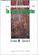Introduction to Theory of Computation (Eitan M. Gurari)

This book explores some of the more important terminologies and questions concerning programs, computers, problems, and computation. The exploration reduces in many cases to a study of mathematical theories, such as those of automata and formal languages; theories that are interesting also in their own right.

•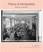Theory of Computation, Making Connections (Jim Hefferon)

The book is geared toward those who thirst for computation theory knowledge. To cater to the demands of a wide range of people, the principles in this book are explained in a way that is easy to understand, digest and apply in the upcoming career.

•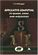Applicative Computing: Its Quarks, Atoms and Molecules

This work covers the advanced topics in main ideas of computing in general. Material is especially useful for the instructor, postgraduate and graduate students of IT-specialties and is suitable for the system of training of specialists.

•Introduction to Soft Computing (Eva Volna)

This book gives an introduction to Soft Computing, which aims to exploit tolerance for imprecision, uncertainty, approximate reasoning, and partial truth in order to achieve close resemblance with human like decision making.

•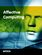Applicative Computing (Jimmy Or)

This book provides an overview of state of the art research in Affective Computing. It presents new ideas, original results and practical experiences in this increasingly important research field.

•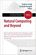Natural Computing and Beyond (Yasuhiro Suzuki, et al)

This book compiles refereed contributions to various aspects of Natural Computing, ranging from computing with slime mold, artificial chemistry, eco-physics, and synthetic biology, to computational aesthetics.

•A Computational Introduction to Number Theory and Algebra

This introductory book emphasises algorithms and applications, such as cryptography and error correcting codes, and is accessible to a broad audience.

•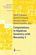Computations in Algebraic Geometry with Macaulay 2 (D.Eisenbud)

This book presents algorithmic tools for algebraic geometry and experimental applications of them using Macaulay 2.

•Common LISP: A Gentle Introduction to Symbolic Computation

This highly accessible introduction to Lisp is suitable both for novices approaching their first programming language and experienced programmers interested in exploring a key tool for artificial intelligence research.

•Logic and Automata: History and Perspectives (Jorg Flum, et al)

This book gives a consolidated overview of the research results achieved in the theory of automata and logic, covers many different facets of logic and automata theory, emphasizing the connections to other disciplines such as games, algorithms, etc.

•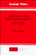Logics of Time and Computation (Robert Goldblatt)

This is a short but excellent introduction to modal, temporal, and dynamic logic, etc. It manages to cover, in highly readable style, the basic completeness, decidability, and expressability results in a variety of logics of the three kinds considered.

•Logic for Computer Science: Automatic Theorem Proving

This book introduces mathematical logic with an emphasis on proof theory and procedures for algorithmic construction of formal proofs. It is useful for the formalization of proofs and basics of automatic theorem proving.

•Theory of Computation and Computing

This is the previous page of Theory of Computation and Computing, we are in the processing to convert all the books there to the new page. Please check this page again!!!

Book Categories
 :All CategoriesRecent BooksMiscellaneous BooksComputer LanguagesComputer ScienceData Science/DatabasesElectrical EngineeringJava and Java EE (J2EE)Linux and UnixMathematicsMicrosoft and .NETMobile ComputingNetworking and CommunicationsSoftware EngineeringSpecial TopicsWeb Programming
Other Categories